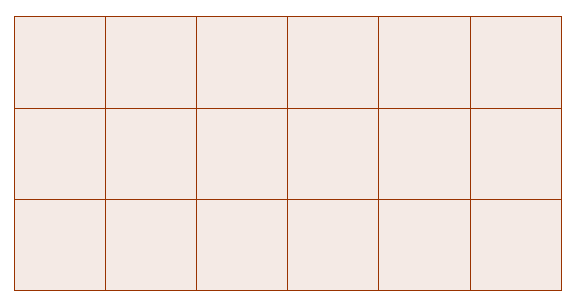This problem follows on from some of the ideas in Tessellating Triangles.

In this problem we're going to be thinking about tessellating different quadrilaterals.

It's quite easy to see how squares tessellate:Have a go at drawing some quadrilaterals, and finding ways to make them tessellate (you can print off some square dotty paper, or some isometric dotty paper, and try drawing different quadrilaterals on it. You could also draw some quadrilaterals using this interactive).

You might want to think about different types of quadrilaterals. For example, can you find a way to tessellate any parallellogram? What about a kite? Or a trapezium?

If your answer is no, give an example of a quadrilateral which doesn't tessellate. Can you explain why it doesn't tessellate?

If your answer is yes, can you explain why all quadrilaterals tessellate, and can you give an algorithm which will produce a tessellation of any quadrilateral?

You might find the interactivity below useful for this:

You can click and drag the corners of the quadrilateral to change its shape.

To produce a tessellation, you can find the midpoint between two points, rotate a shape around a point, and translate a shape by a given vector.

If you enjoyed this problem, why not have a look at Tessellating Hexagons?